## Selection Sort - Data Structures and Algorithms

Selection sort is a simple sorting algorithm. This sorting algorithm is an in-place comparison-based algorithm in which the list is divided into two parts, the sorted part at the left end and the unsorted part at the right end. Initially, the sorted part is empty and the unsorted part is the entire list.

The smallest element is selected from the unsorted array and swapped with the leftmost element, and that element becomes a part of the sorted array. This process continues moving unsorted array boundary by one element to the right.

This algorithm is not suitable for large data sets as its average and worst case complexities are of ?(n2), where n is the number of items.

## 1. How Selection Sort Works

Consider the following depicted array as an example.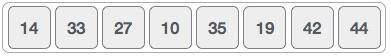For the first position in the sorted list, the whole list is scanned sequentially. The first position where 14 is stored presently, we search the whole list and find that 10 is the lowest value.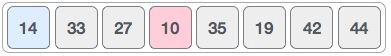So we replace 14 with 10. After one iteration 10, which happens to be the minimum value in the list, appears in the first position of the sorted list.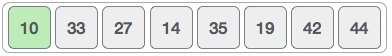For the second position, where 33 is residing, we start scanning the rest of the list in a linear manner.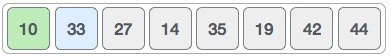We find that 14 is the second lowest value in the list and it should appear at the second place. We swap these values.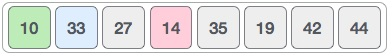After two iterations, two least values are positioned at the beginning in a sorted manner.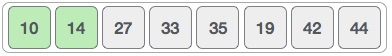The same process is applied to the rest of the items in the array.

Following is a pictorial depiction of the entire sorting process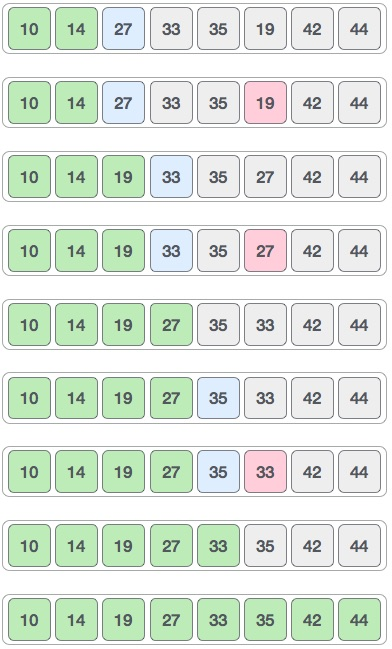Now, let us learn some programming aspects of selection sort.

### 2. Algorithm

Step 1 − Set MIN to location 0

Step 2 − Search the minimum element in the list

Step 3 − Swap with value at location MIN

Step 4 − Increment MIN to point to next element

Step 5 − Repeat until list is sorted

### 3. Pseudocode

procedure selection sort

list  : array of items

n     : size of list

for i = 1 to n - 1

/* set current element as minimum*/

min = i

/* check the element to be minimum */

for j = i+1 to n

if list[j] < list[min] then

min = j;

end if

end for

/* swap the minimum element with the current element*/

if indexMin != i  then

swap list[min] and list[i]

end if

end for

end procedure

New
Related